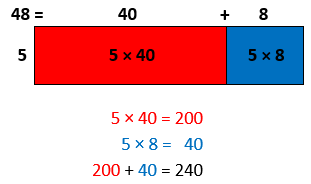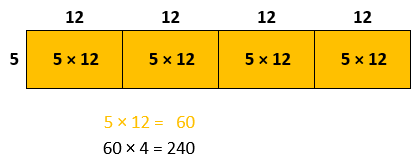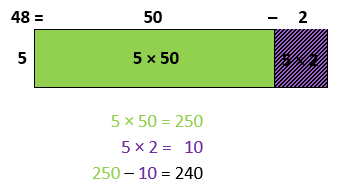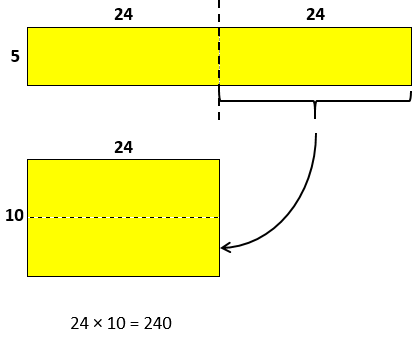# Multi-Digit Multiplication

## Objective

Multiply two-, three-, and four-digit numbers by one-digit numbers using a variety of mental strategies.

## Common Core Standards

### Core Standards

?

• 4.NBT.B.5 — Multiply a whole number of up to four digits by a one-digit whole number, and multiply two two-digit numbers, using strategies based on place value and the properties of operations. Illustrate and explain the calculation by using equations, rectangular arrays, and/or area models.

?

• 3.NBT.A.3

• 4.NBT.A.1

• 4.NBT.B.4

• 3.OA.B.5

• 3.OA.C.7

## Criteria for Success

?

1. Multiply two-, three-, and four-digit numbers by one-digit numbers using a variety of mental strategies, including:
1. Finding partial products and adding them together (e.g., 48 x 5 = (40 + 8) x 5 = (40 x 5) + (8 x 5))
2. Finding a benchmark or “friendly” number and adjusting from there (e.g., 48 x 5 = (50 – 2) x 5 = (50 x 5) – (2 x 5))
3. Doubling and halving (e.g., 48 x 5 = 24 x 10), and
4. Breaking factors into smaller factors (e.g., 5 x 48 = 5 x 12 x 4).
2. Understand and explain why various mental strategies work (MP.3).

## Tips for Teachers

?

• As Bill McCallum notes on his blog Mathematical Musings, “the distinction between a strategy and an algorithm is that an algorithm is general, it works in all possible cases, whereas a strategy might be specialized." (Algorithms Grades 2-5, Mathematical Musings)  He goes on to say that the progression toward fluency with any computation begins with strategies, then algorithms (note the plurality), then the standard algorithm. Thus, this lesson attempts to explore strategies students may use for particular computational problems before they jump into generalizable methods and algorithms in later lessons.
• The first page of the Problem Set provides an array of Number Talks, similar to Anchor Task #2. You may decide to use these tasks in a similar way to Anchor Task #2, using them as a whole class and restricting the use of paper and pencil, forcing the use of mental math to solve and therefore encouraging the use of the mental strategies discussed in the lesson. Or, if Anchor Tasks #1 and #2 take up much of the lesson block, you can use them as a source for problems to be spread out over various parts of the day in the future so that students can gradually develop the use of each strategy.
• Similar to the Problem Set, the first page of the Homework provides an array of Number Talks, similar to Anchor Task #2. You may decide to send students home with instructions for guardians about how to have a Number Talk with their child. Or, you could save the first page as a source for problems to be spread out over various parts of the day in the future so that students can gradually develop the use of each strategy.

#### Remote Learning Guidance

If you need to adapt or shorten this lesson for remote learning, we suggest prioritizing Anchor Task 1 (benefits from discussion). Find more guidance on adapting our math curriculum for remote learning here.

#### Fishtank Plus

• Problem Set
• Student Handout Editor
• Vocabulary Package

?

### Problem 1

Four different students solved the problem 48 x 5 below. Study their work and determine whether the strategy they used works.

 Student AStudent BStudent CStudent D### Problem 2

 3 x 2 3 x 60 3 x 400 3 x 462 6 x 50 6 x 300 6 x 350 6 x 349 50 x 2 x 9 2 x 9 x 50 2 x 450 500 x 2 250 x 4 125 x 8

#### References

Book: Number Talks: Helping Children Build Mental Math and Computation Strategies, Grades K-5 by Sherry Parrish (Math Solutions, 2010)

## Problem Set & Homework

#### Discussion of Problem Set

• What was the best option for #2? Were there other options that were decent options but not the best option? How did you decide which was the better option of the two?
• Were there specific strategies that you found yourself relying on repeatedly in #3?
• Which strategies seem to be useful for all computations? Which ones seem to be useful just in specific cases?

?

Solve. Show or explain your work.

1.   35 x 6
2.   5 x 246
3.   1,199 x 3

### Mastery Response

?